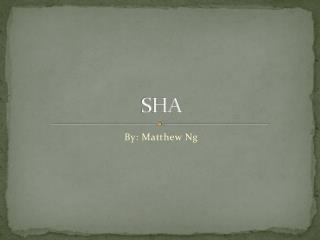DownloadDownload PresentationSHA

# SHA

Télécharger la présentation## SHA

- - - - - - - - - - - - - - - - - - - - - - - - - - - E N D - - - - - - - - - - - - - - - - - - - - - - - - - - -
##### Presentation Transcript

1. SHA By: Matthew Ng

2. Operations that SHA1 can perform • AND, XOR, OR, Complement, Circular Left shift, and Addition Modulo • Circular Left Shift is done with s positions (0 ≤ s ≤ 31) – Denoted by ROTLs • Addition Modulo is 2 32

3. SHA-1-Pad • This is pads the input. • The binary representation of |x| is l and it is at most 64 bits • If |l| < 64 bits then it is padded from the left with zeros to make 64 bits. • In the construction of y – one is appended to x and then concatenated with enough zeros so the length is congruent to 448 modulo 512. • A concatenation 64 bits that contain binary representation of the original length of x. • Divide the string by 512 and create a concatenation of n blocks each of them being 512 bits. • y = M1 || M2 || … || Mn

4. SHA-1-pad (x) : • D = (447 - |x|) Mod 512 • L = binary of |x| where |l| = 64 • y = x || 1 || 0d || 1 • |x| ≤ 264 - 1

5. SHA-1 Algorithm (one round) • ABCDE are 32-bit words in the state • F is a non-linear function that varies • <<<n is a left bit rotation by n places (n varies for each operation) • Wt is the expanded message word of round t • Kt is the round constant of round t • Boxes addition modulo 232

6. Let’s look at function f • The function is based on what round it is on. • If the round is between 0 and 19 • F(B,C,D) : (B ∧C) ∨((~B) ∧ D) • If the round is between 20 and 39 • F(B,C,D): (B XOR C XOR D) • If the round is between 40 to 59 • F(B,C,D): (B ∧C) ∨(B ∧ D) ∨(C ∧ D) • If the round is between 60 to 79 • F(B,C,D): (B XOR C XOR D)

7. Keys • The keys also depend on what the round is • When the round is between 0 to 19 • Kt = 5A827999 • When the round is between 20 to 39 • Kt = 6ED9EBA1 • When the round is between 40 to 59 • Kt = 8F1BBCDC • When the round is between 60 to 79 • Kt = CA62C1D6

8. Algorithm y = SHA-1-Pad(x) //y = M1 || M2 || .. || Mn //Initialize Variables H0 = 67452301 H1 = EFCDAB89 H2 = 98BADCFE H3 = 10325476 H4 = CSD2E1F0 For i= 1 to n Mi = W0 || W1 || … || W15 //Wi is a word For t = 16 to 79 Wt = ROTL1 (Wt-3 XOR Wt-8 XOR Wt-14 XOR Wt-16)

9. Algorithm Continued A = H0 , B = H1, C = H2, D = H3, E = H4 For t = 0 to 79 temp = ROTL5(A) + Ft(B,C,D) + E + Wi + Kt E = D, D = C, C = ROTL30(B), B = A, A = temp H0 = H0 + A H1 = H1 + B H2 = H2 + C H3 = H3 + D H4 = H4 + E Return (H0 || H1 || H2 || H3 || H4)

10. References • Stinson Textbook